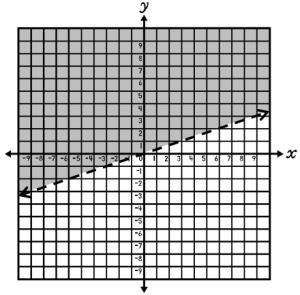# Let's Get Started

Let's practice how to graph one-variable and two-variable inequalities and how to determine the symbolic representation of an inequality given its graph.

TEKS Standards and Student Expectations

A(2) Describing and graphing linear functions, equations, and inequalities. The student is expected to:

A(2)(H) write linear inequalities in two variables given a table of values, a graph, and a verbal description

A(3) Writing and solving linear functions, equations, and inequalities. The student is expected to:

A(3)(D) graph the solution set of linear inequalities in two variables on the coordinate plane

Resource Objective(s)

Given the graph of an inequality, students will write the symbolic representation of the inequality.

Essential Questions

What is a solution to an inequality?

How do you graph a one-variable or two-variable inequality?

What is the inequality symbol if the line is dotted?

What is the inequality symbol if the line is solid?

What is the inequality symbol if the the shading is above the line?

What is the inequality symbol if the the shading is below the line?

Vocabulary

• Solution
• Included
• Excluded

# Graphing Solutions to Inequalities in One Variable

A solution of an inequality is any value that makes the inequality true.

For inequalities in one variable, such as x ≤ 2, solutions are graphed on a number line.

For the inequality, x ≤ 2, the solutions are graphed by drawing a closed circle at 2 and shading to the left on the number line. This indicates that all real numbers less than or equal to 2 are solutions.

Some specific examples of solutions to the inequality include -10, -8, -1, 0, and 1. But keep in mind, the set of solutions to the inequality include all real numbers less than or equal to 2.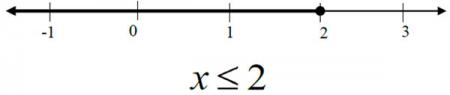For the inequality, x

Some specific examples of solutions to the inequality include -5, 1, 2, 3, and 7. In this case the set of solutions to the inequality include all real numbers less than 8.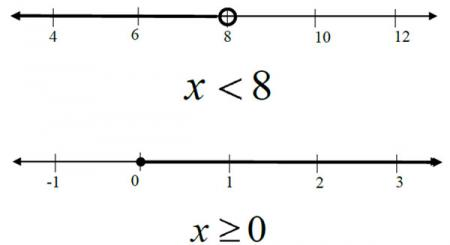For the inequality x ≥ 0 the solutions are graphed by drawing a closed circle at 0 and shading to the right on the number line. This indicates that all real numbers greater than or equal to 0 are solutions to the inequality.

Some specific examples of solutions to the inequality include 1, 2, 3, 7, and 10.5. The set of solutions to the inequality include all real numbers greater than or equal to 0.

# Graphing Solutions to Inequalities in Two Variables

To graph inequalities in two variables, you must do the following:

1. Make sure the inequality is solved for y. For example: y ≤ 2/3x + 1.

2. Graph the equation of the line for the inequality.

3. The line of the graph will depend on the symbol. If it is "greater than" or "less than" you will use > or <Use a dashed line with the greater than (>) or less than (<) symbols. The ordered pairs on the dashed line are NOT a part of the solution.

4. If it is If it is "greater than or equal to" or "less than or equal to" you will use  or Use a solid line with the greater than or equal to (≥) or less than or equal to (≤) symbols. The ordered pairs on the solid line are a part of the solution.

5. Choose an ordered pair on both sides of the graphed line.

6. Substitute the ordered pair into the original inequality to determine which part or side of the line should be shaded.

7. Shade the side of the graph that yields the correct solution when the ordered pair is substituted.

# Example 1: Graphing Solutions to Inequalities in Two Variables

For inequalities in two variables, such as ≤ 2/3x + 1, solutions are graphed on a coordinate plane.

For the inequality ≤ 2/3x + 1, the solutions are graphed by drawing a solid line at = 2/3x + 1 (y-intercept at 1 and slope of 2/3) and shading below the line. This indicates that all real numbers on the line and in the shaded area are solutions to the inequality.

Some specific examples of solutions to the inequality include (0, 0), (8, -2), and (1, -2.5).

Keep in mind that the set of solutions to the inequality include all real numbers on the line = 2/3x +1 yields and in the shaded area below the line.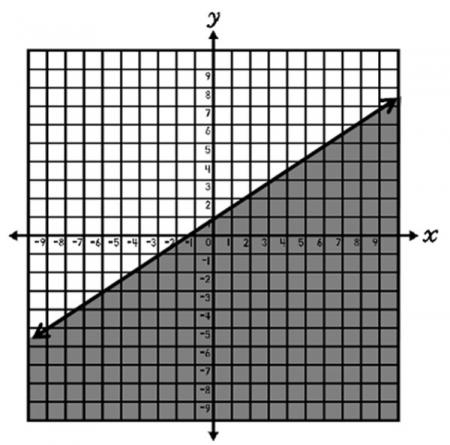> ≤ ≥ Dashed Dashed Solid Solid

A solid line is used for inequalities that include (less than or equal to) and  (greater than or equal to) since the solutions are included on the graphed line. For example, in the inequality ≤ 2/3x + 1, the solutions are both on the line = 2/3x + 1 creates, as well as below this line. The point (0, 1), which lies on the line = 2/3x + 1, makes the inequality y ≤ 2/3x + 1 true. So a solid line, which includes all solutions, is drawn to indicate a boundary.

# Example 2: Graphing Solutions to Inequalities in Two Variables

For the inequality > 1/3x – 2, the solutions are graphed by drawing a dashed line at y= 1/3x –2 (y-intercept at -2 and slope of 1/3) and shading above the line. This indicates that all real numbers in the shaded area, but not those on the line, are solutions to the inequality.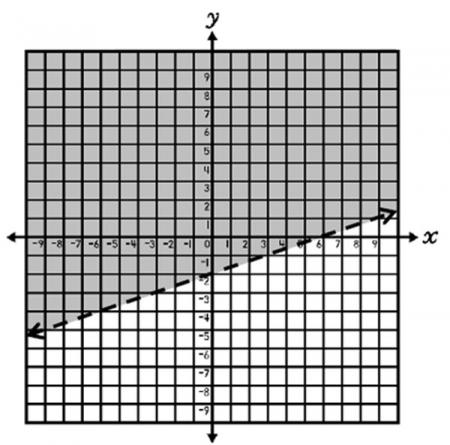> ≤ ≥ Dashed Dashed Solid Solid

A dashed line is used for inequalities including  (less than) and > (greater than) since the solutions are not included on the graphed line. For example, in the inequality > 1/3x – 2, the solutions are not on the graphed line, only above. The point (0, -2), which lies on the line = 1/3x – 2, does not make the inequality true. A dashed line is drawn to indicate a boundary for the solutions.

# Additional Examples: Graphing Solutions to Inequalities in Two Variables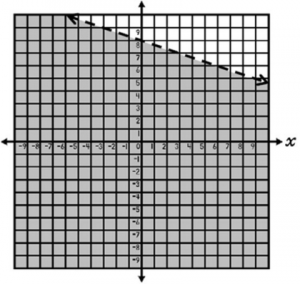y x + 8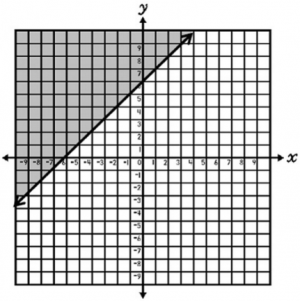y ≥ x + 6

# Interactive Linear Inequality

Click on the following link: Linear Inequality Activity

Adjust the graph by clicking and dragging point A or point C, and the inequality will adjust accordingly.  To change the sign, click on the inequality itself.

Adjust the graph on the website to match the following graphs.  Then write the corresponding inequalities in your notes.

1.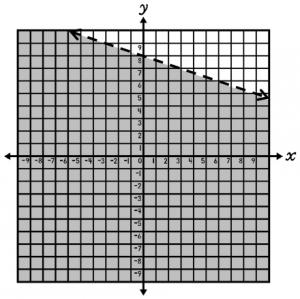2.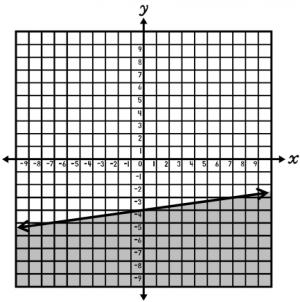3.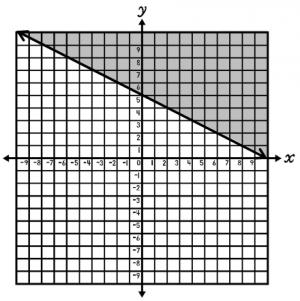4.